# RS Aggarwal Solutions For Class 7 Maths Exercise 1A Chapter 1 Integers

The pdf of RS Aggarwal Solutions for the Exercise 1A of Class 7 Maths Chapter 1, Integers are available here. BYJU’S experts have solved the questions present in the Exercise 1A in Maths, and this will help the students in solving the problems without any error. As this is the first exercise, this will help the students to understand the basic concepts of integers as well. By practising the RS Aggarwal Solutions for Class 7, students will be able to score good marks in Maths.

## Download the PDF of RS Aggarwal Solutions For Class 7 Maths Chapter 1 Integers – Exercise 1A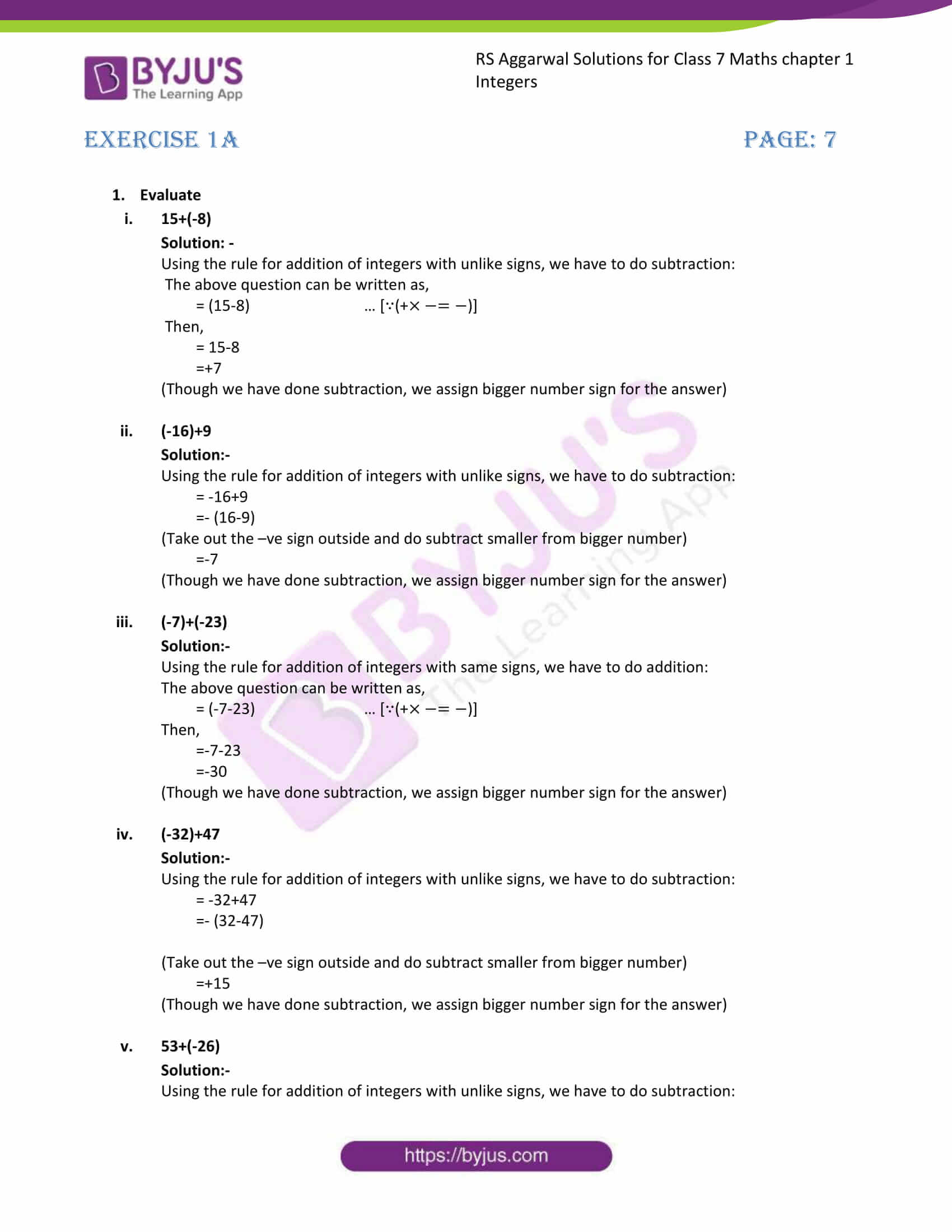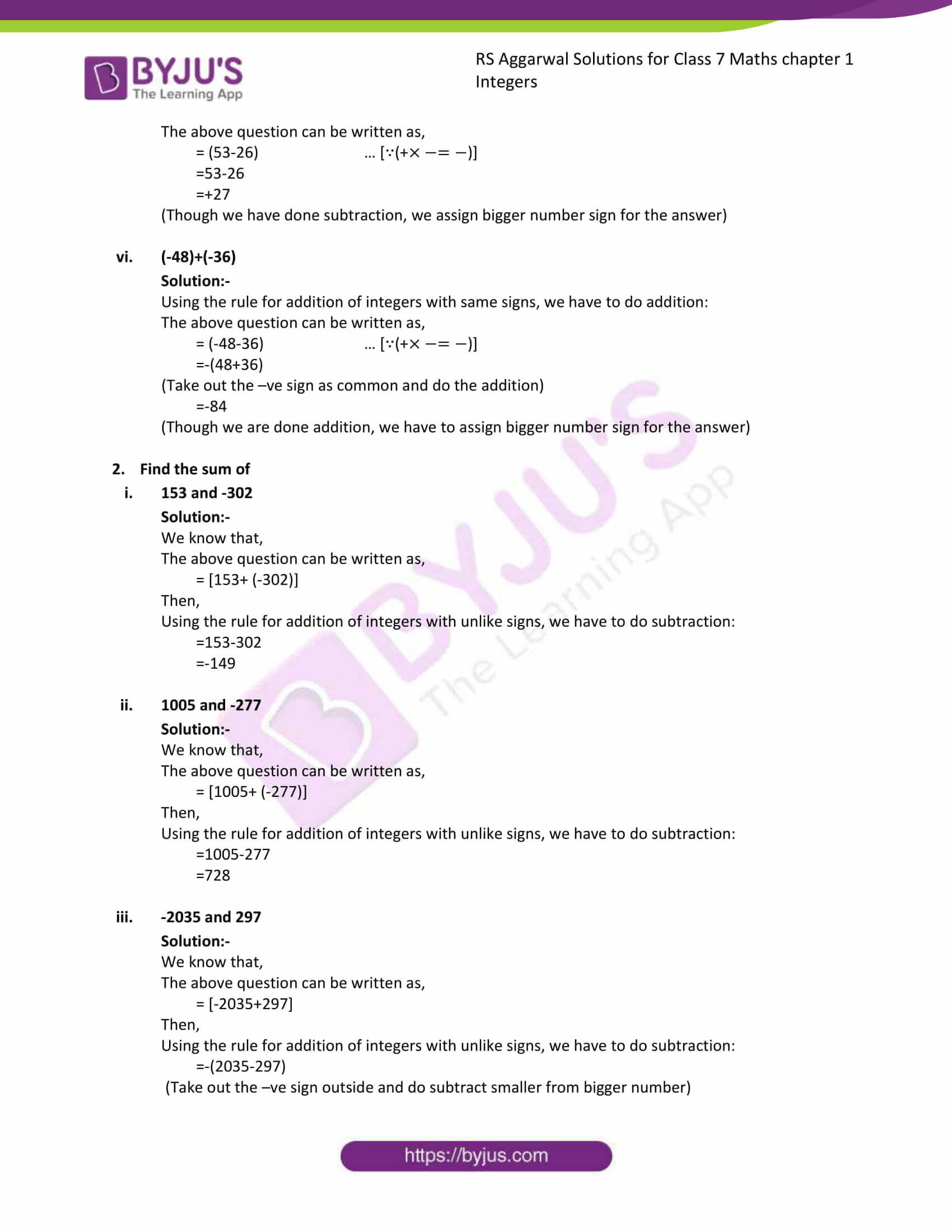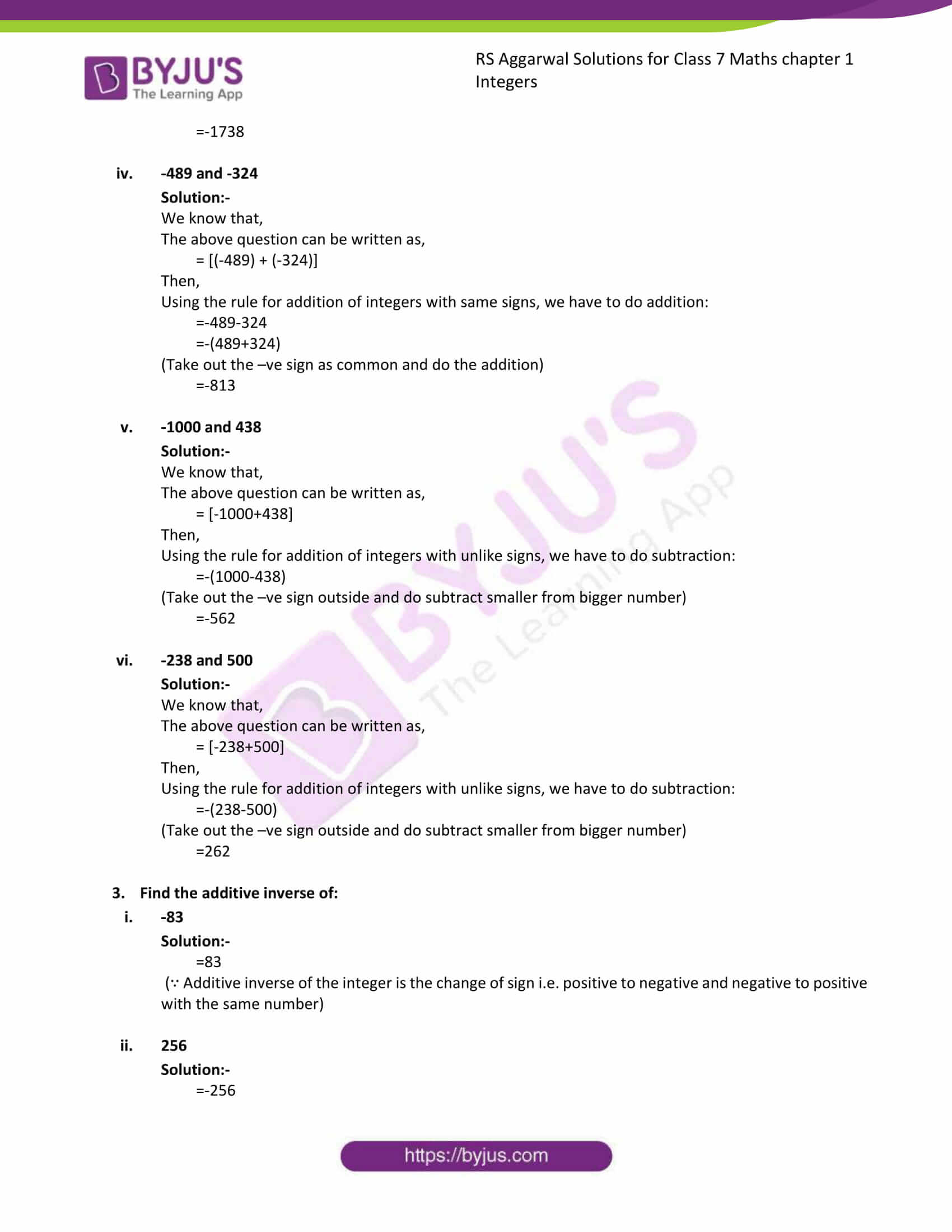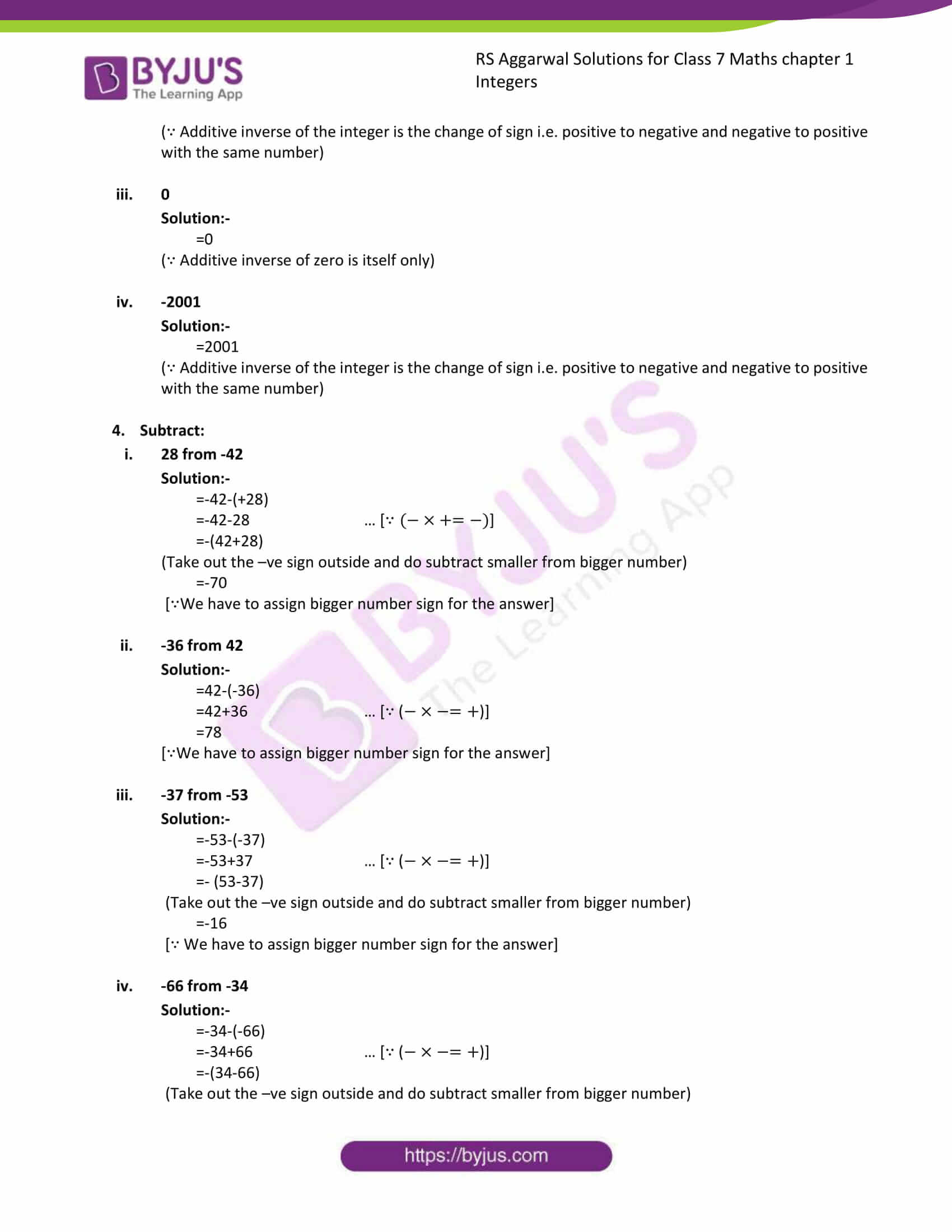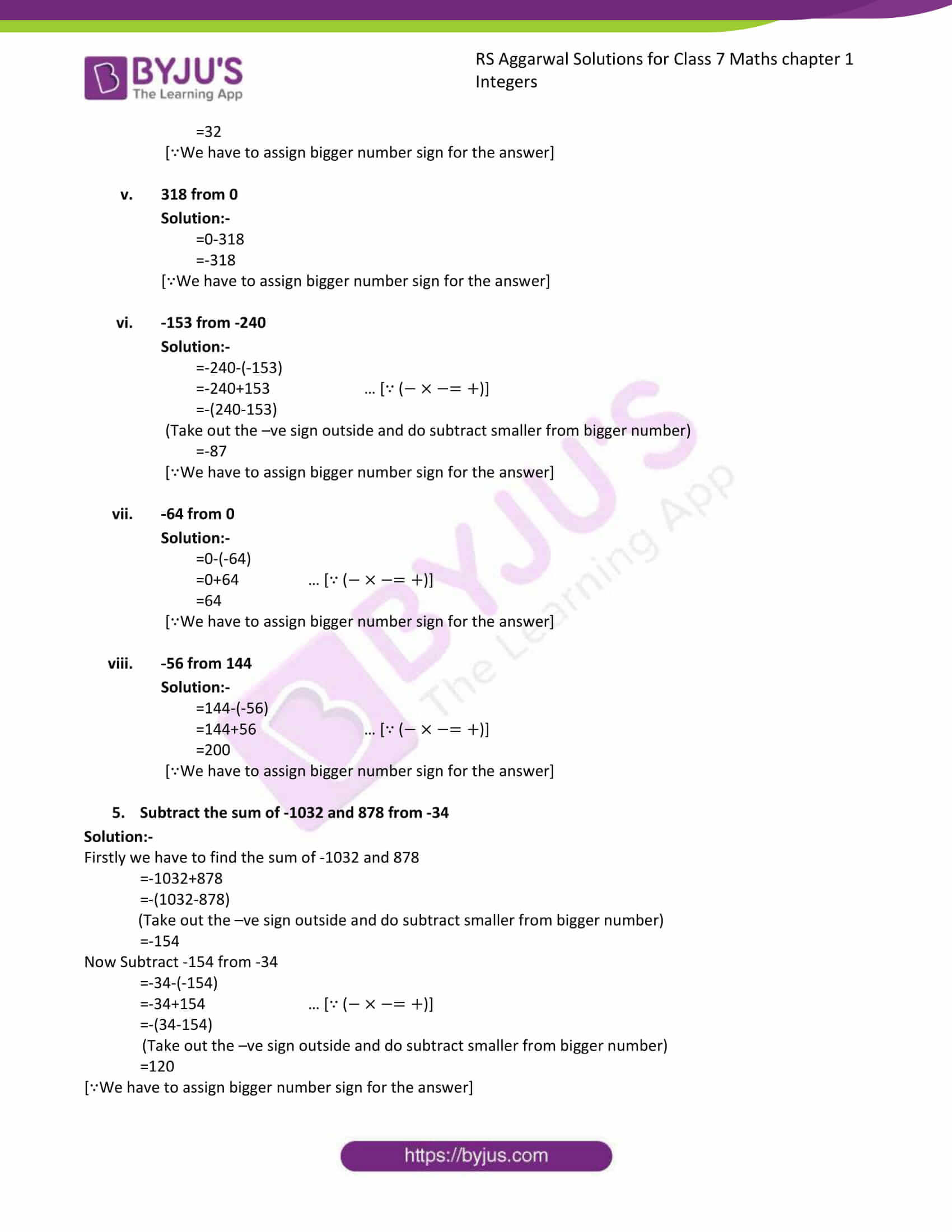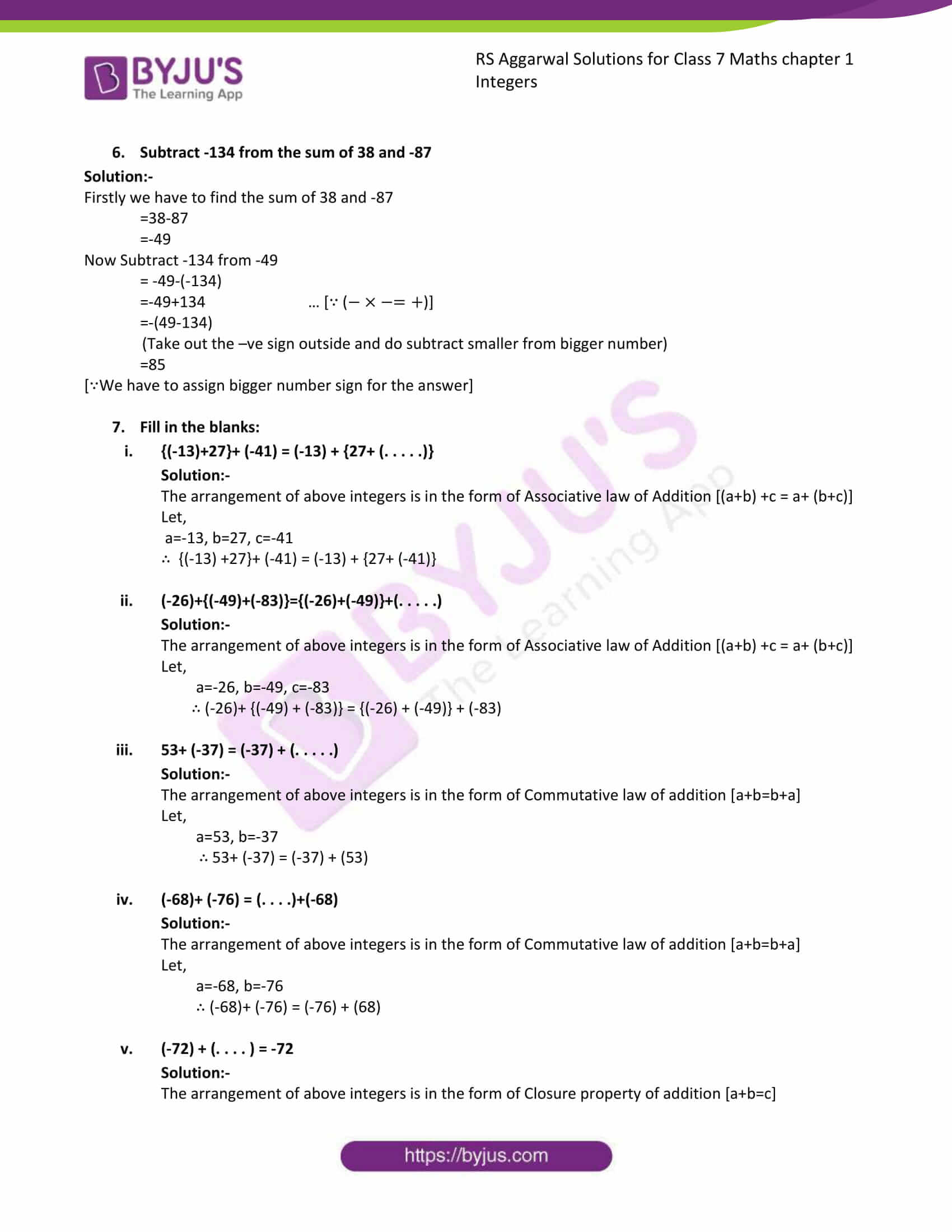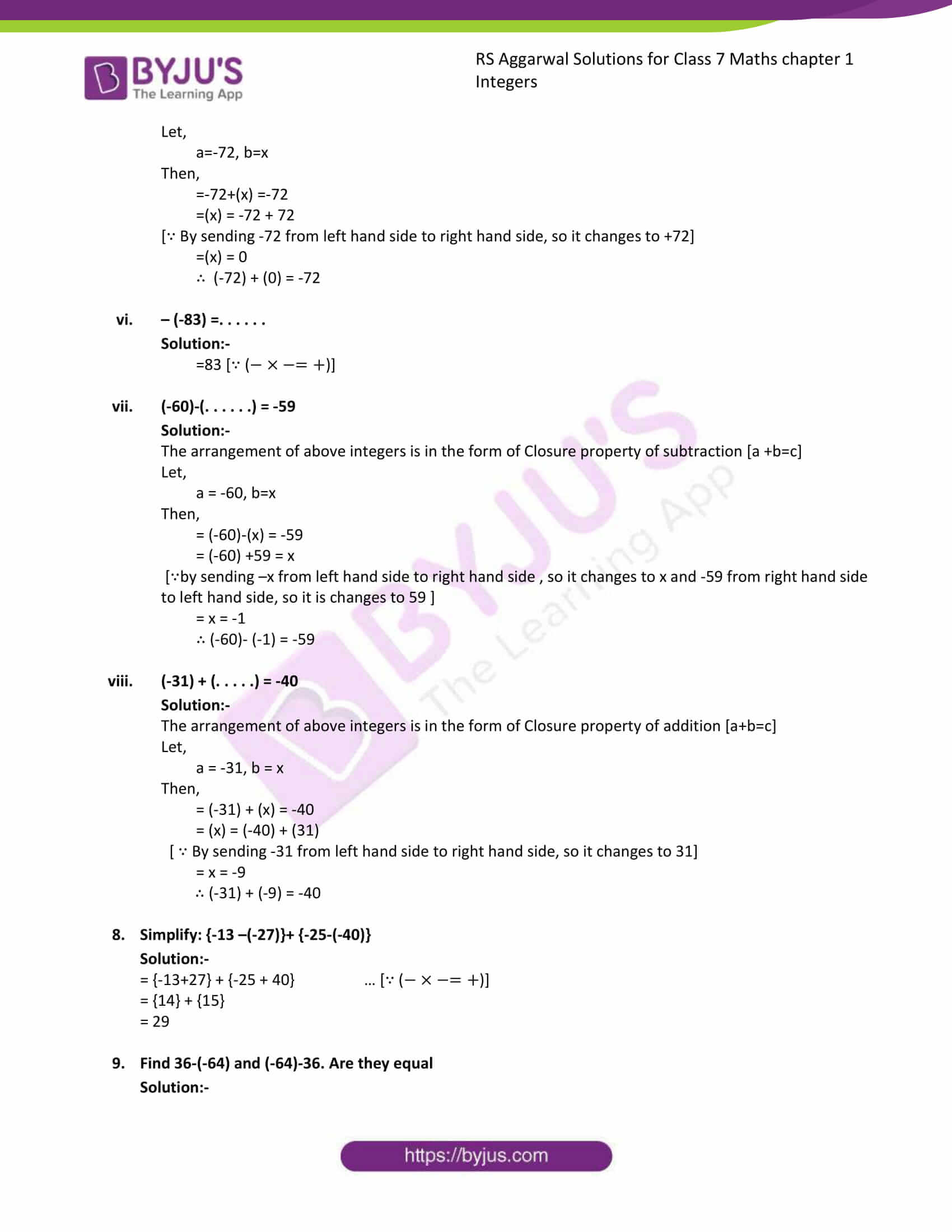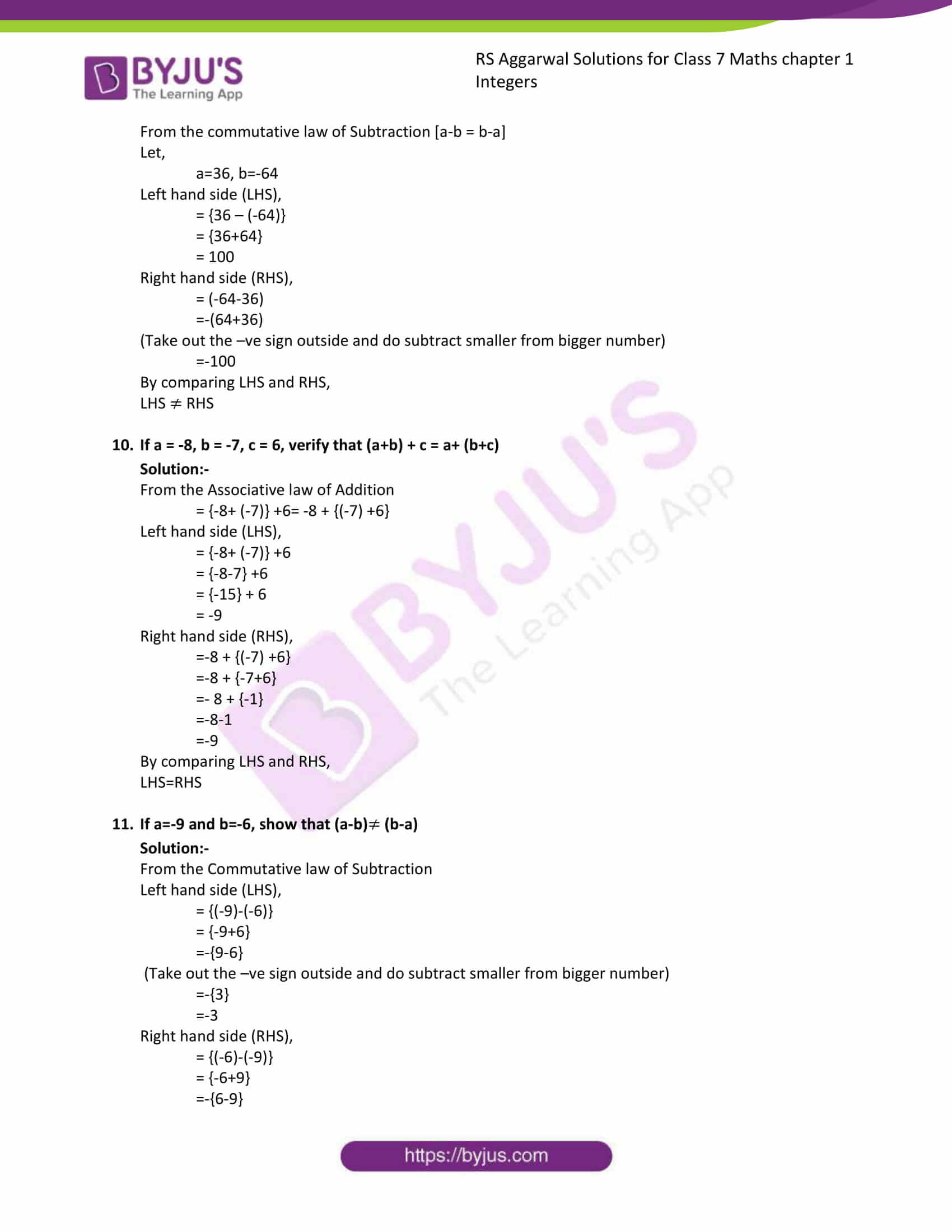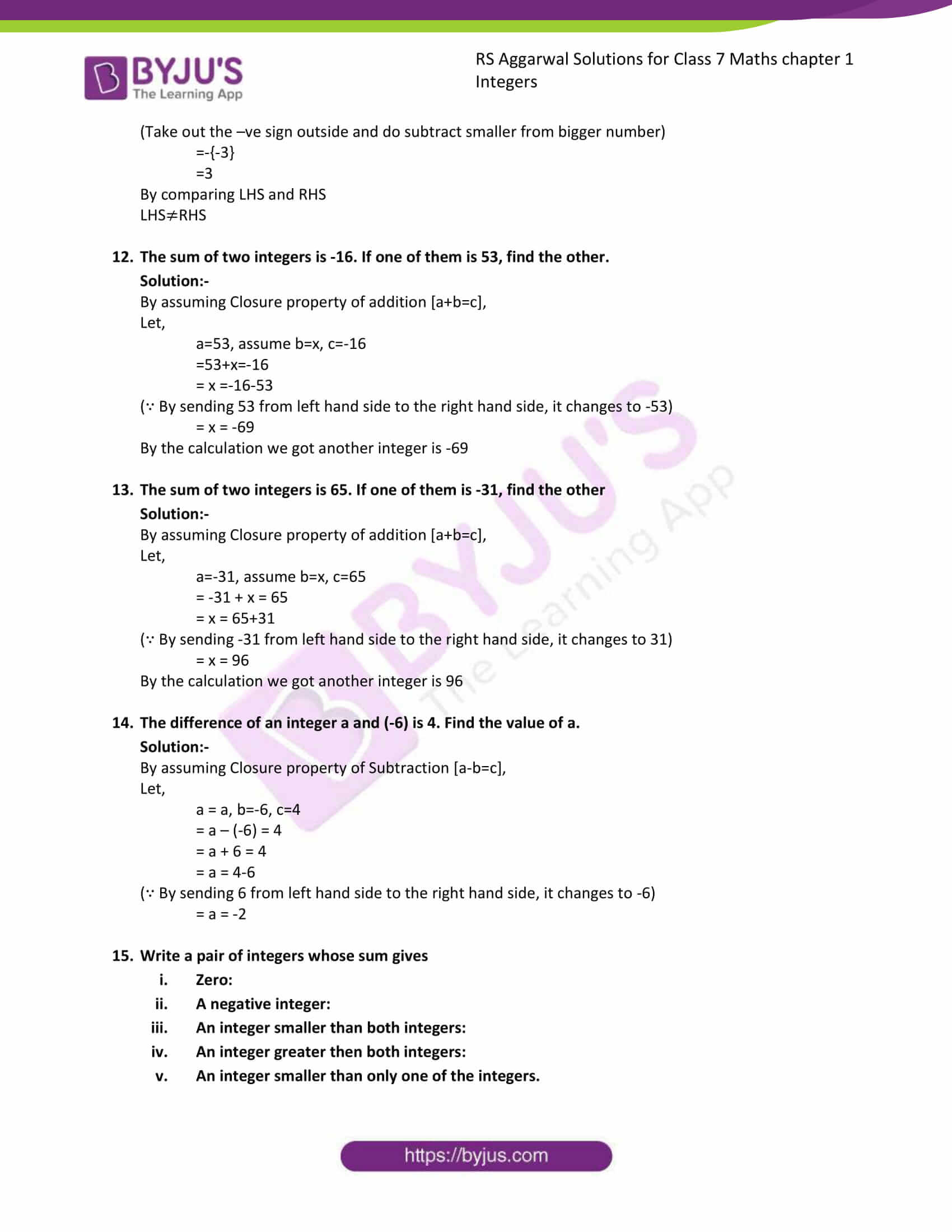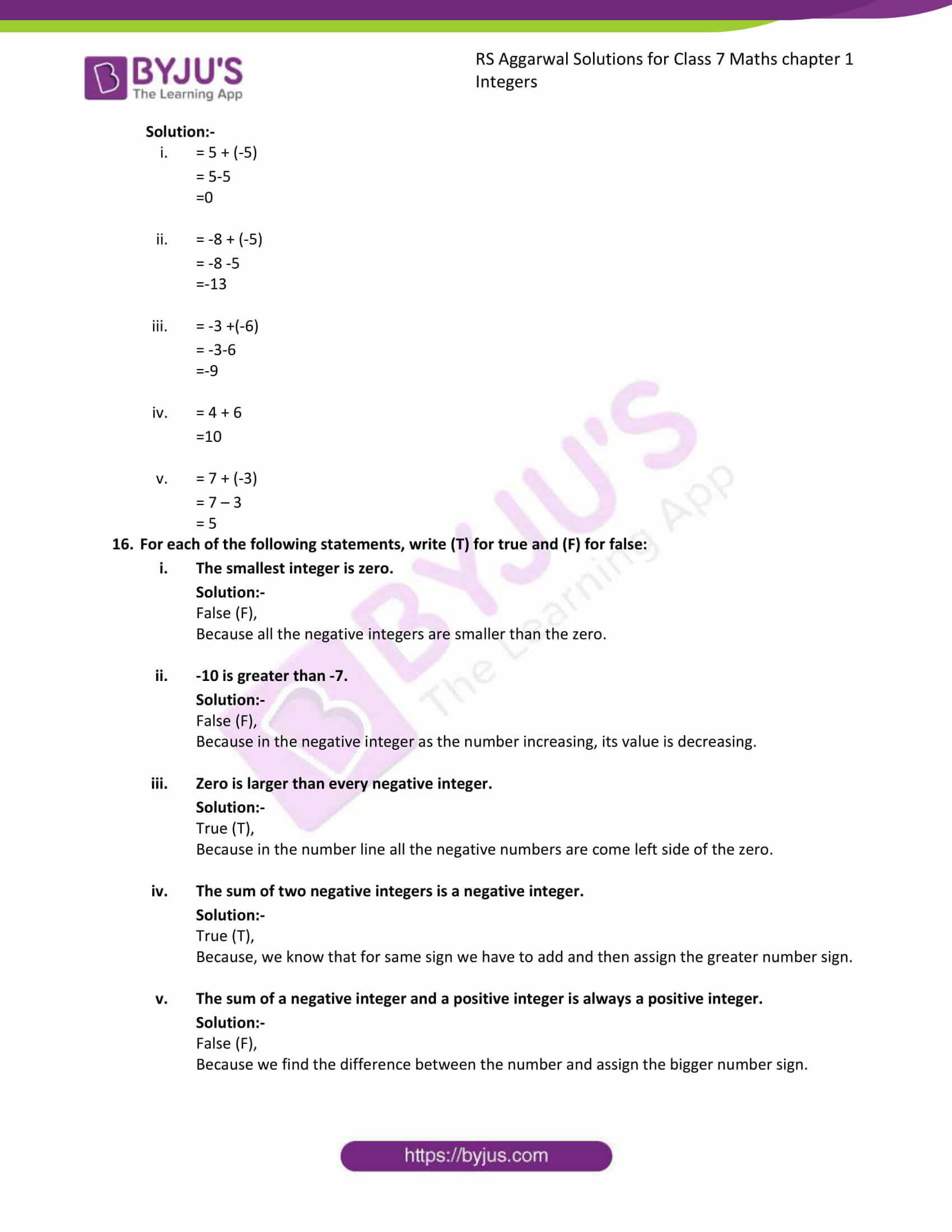### Access answers to Maths RS Aggarwal Solutions for Class 7 Chapter 1 – Integers Exercise 1A

1. Evaluate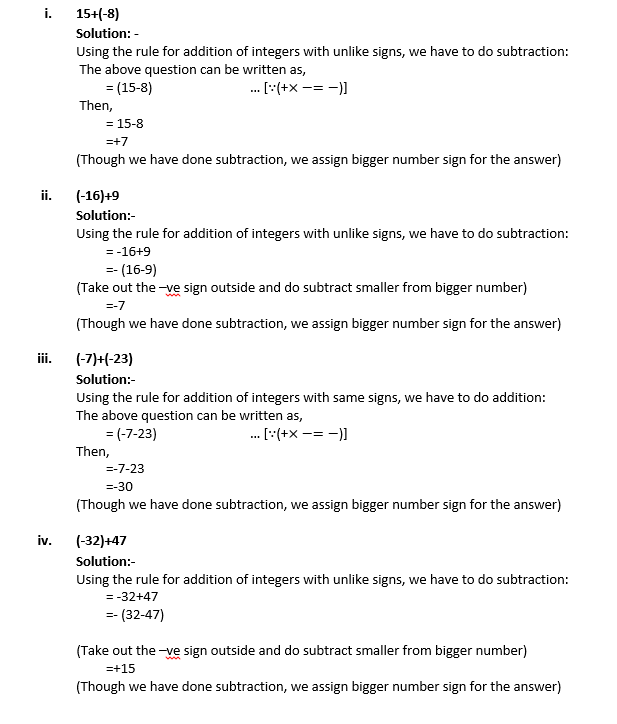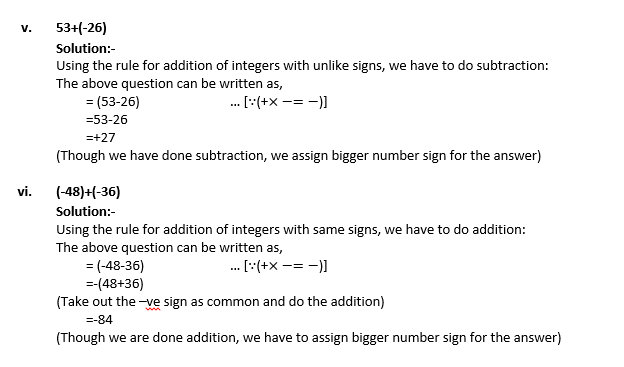2. Find the sum of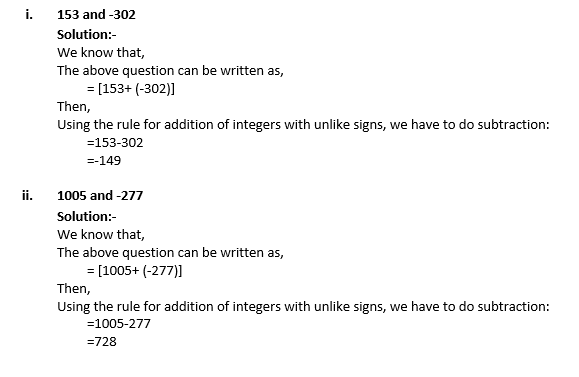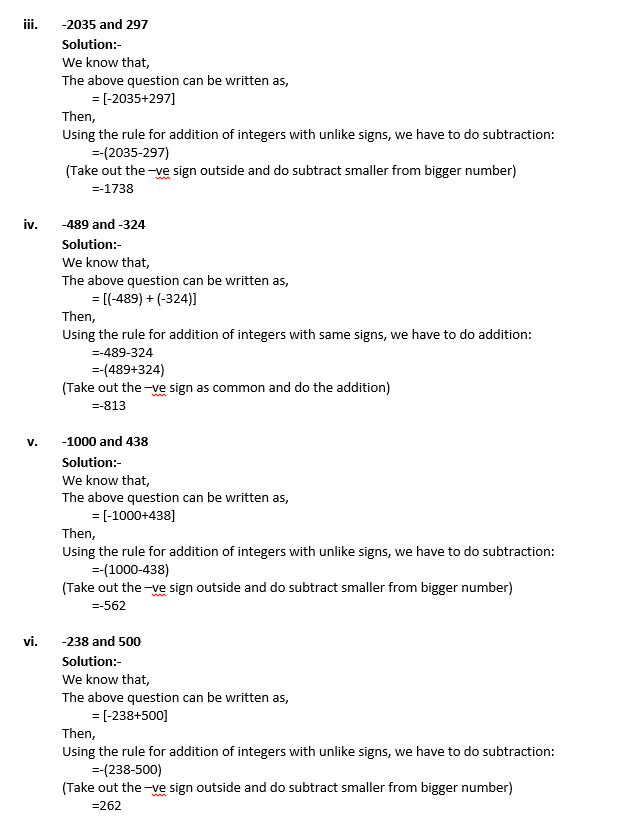3. Find the additive inverse of: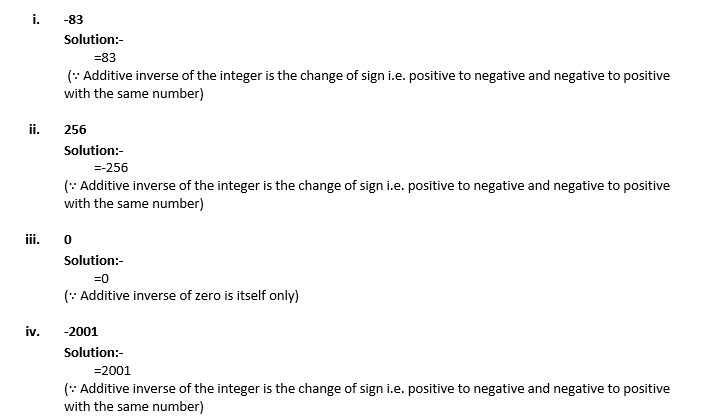4. Subtract: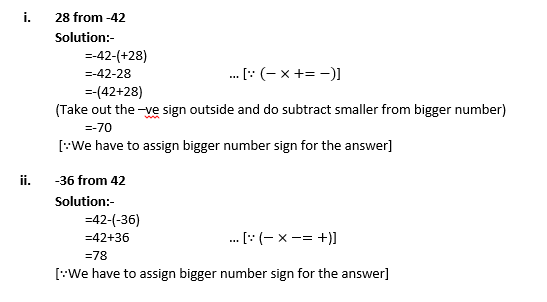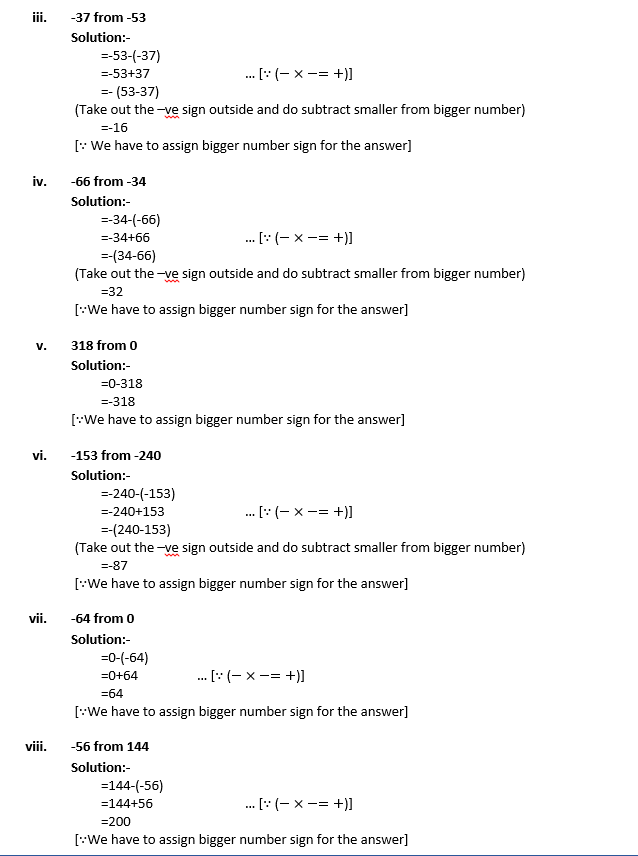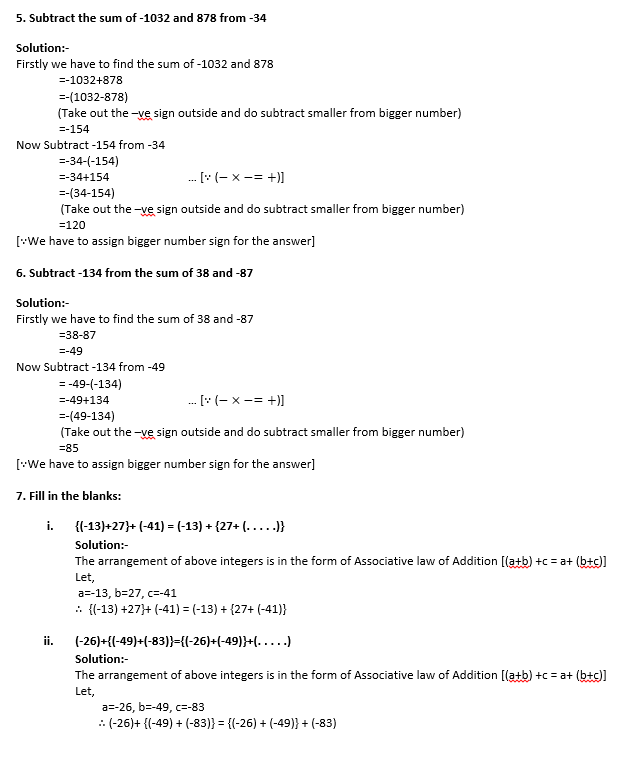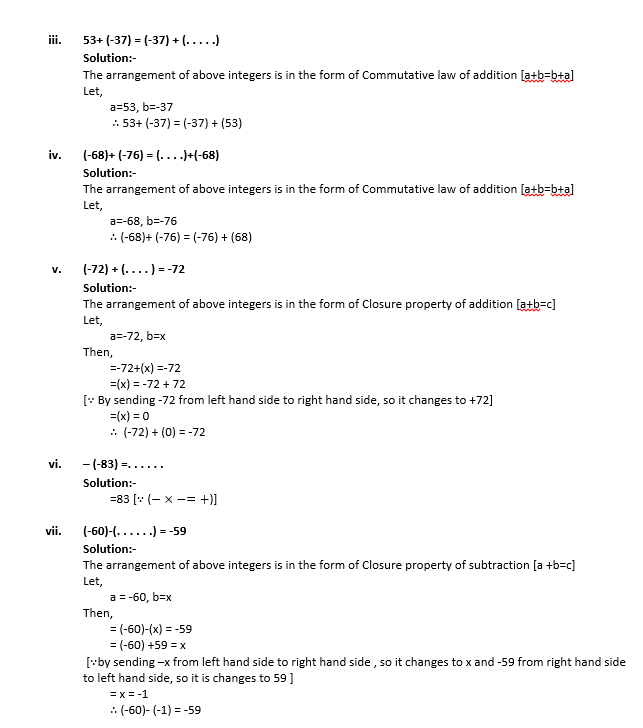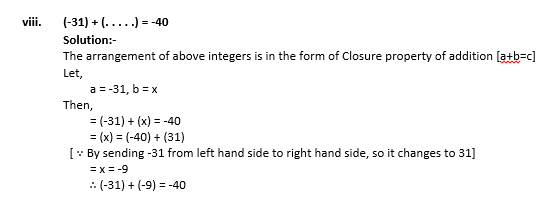8. Simplify: {-13 –(-27)}+ {-25-(-40)}

Solution:-

= {-13+27} + {-25 + 40} …[∴( – x – = + ) ]

= {14} + {15}

= 29

9. Find 36-(-64) and (-64)-36. Are they equal

Solution:-

From the commutative law of Subtraction [a-b = b-a]

Let,

a=36, b=-64

Left hand side (LHS),

= {36 – (-64)}

= {36+64}

= 100

Right hand side (RHS),

= (-64-36)

=-(64+36)

(Take out the –ve sign outside and do subtract smaller from bigger number)

=-100

By comparing LHS and RHS,

LHS ≠RHS

10. If a = -8, b = -7, c = 6, verify that (a+b) + c = a+ (b+c)

Solution:-

From the Associative law of Addition

= {-8+ (-7)} +6= -8 + {(-7) +6}

Left hand side (LHS),

= {-8+ (-7)} +6

= {-8-7} +6

= {-15} + 6

= -9

Right hand side (RHS),

=-8 + {(-7) +6}

=-8 + {-7+6}

=- 8 + {-1}

=-8-1

=-9

By comparing LHS and RHS,

LHS=RHS

11. If a=-9 and b=-6, show that (a-b) ≠ (b-a)

Solution:-

From the Commutative law of Subtraction

Left hand side (LHS),

= {(-9)-(-6)}

= {-9+6}

=-{9-6}

(Take out the –ve sign outside and do subtract smaller from bigger number)

=-{3}

=-3

Right hand side (RHS),

= {(-6)-(-9)}

= {-6+9}

=-{6-9}

(Take out the –ve sign outside and do subtract smaller from bigger number)

=-{-3}

=3

By comparing LHS and RHS

LHS≠RHS

12. The sum of two integers is -16. If one of them is 53, find the other.

Solution:-

By assuming Closure property of addition [a+b=c],

Let,

a=53, assume b=x, c=-16

=53+x=-16

= x =-16-53

( By sending 53 from left hand side to the right hand side, it changes to -53)

= x = -69

By the calculation we got another integer is -69

13. The sum of two integers is 65. If one of them is -31, find the other

Solution:-

By assuming Closure property of addition [a+b=c],

Let,

a=-31, assume b=x, c=65

= -31 + x = 65

= x = 65+31

( By sending -31 from left hand side to the right hand side, it changes to 31)

= x = 96

By the calculation we got another integer is 96

14. The difference of an integer a and (-6) is 4. Find the value of a.

Solution:-

By assuming Closure property of Subtraction [a-b=c],

Let,

a = a, b=-6, c=4

= a – (-6) = 4

= a + 6 = 4

= a = 4-6

( By sending 6 from left hand side to the right hand side, it changes to -6)

= a = -2

15. Write a pair of integers whose sum gives

(i) Zero:
(ii) A negative integer:
(iii) An integer smaller than both integers:
(iv) An integer greater then both integers:
(v) An integer smaller than only one of the integers.

Solution:-

(i) = 5 + (-5)

= 5-5

=0

(ii) = -8 + (-5)

= -8 -5

=-13

(iii) = -3 +(-6)

= -3-6

=-9

(iv) = 4 + 6

=10

(v) = 7 + (-3)

= 7 – 3

= 5

16. For each of the following statements, write (T) for true and (F) for false:

(i) The smallest integer is zero.

Solution:-

False (F),

Because all the negative integers are smaller than the zero.

(ii) -10 is greater than -7.

Solution:-

False (F),

Because in the negative integer as the number increasing, its value is decreasing.

(iii) Zero is larger than every negative integer.

Solution:-

True (T),

Because in the number line all the negative numbers are come left side of the zero.

(iv) The sum of two negative integers is a negative integer.

Solution:-

True (T),

Because, we know that for same sign we have to add and then assign the greater number sign.

(v) The sum of a negative integer and a positive integer is always a positive integer.

Solution:-

False (F),

Because we find the difference between the number and assign the bigger number sign.

### RS Aggarwal Solutions for Class 7 Maths Exercise 1A Chapter – 1 Integers

RS Aggarwal Solutions For Class 7 Maths Chapter 1 Integers Exercise 1A has problems which are based on the addition of Integers, subtraction of Integers, properties of addition of integers and properties of subtraction of integers. Students are suggested to try solving the questions from RS Aggarwal Solutions book of Class 7 and then refer to these solutions to know the best way of approaching the different questions.